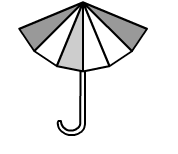# An umbrella is made by stitching 12 triangular pieces of cloth, each measuring

Question:

An umbrella is made by stitching 12 triangular pieces of cloth, each measuring (50 cm × 20 cm × 50 cm). Find the area of the cloth used in it.Solution:

We know that the triangle is an isosceles triangle.
Thus, we can find out the area of one triangular piece of cloth.

Area of isosceles triangle $=\frac{b}{4} \sqrt{4 a^{2}-b^{2}}$

$=\frac{20}{4} \times \sqrt{4(50)^{2}-20^{2}} \quad(a=50 \mathrm{~cm}$ and $b=20 \mathrm{~cm})$

$=5 \times \sqrt{10000-400}$

$=5 \times \sqrt{9600}$

$=5 \times 40 \sqrt{6}$

$=200 \sqrt{6}$

$=490 \mathrm{~cm}^{2}$

Now,
Area of 1 triangular piece of cloth = 490 cm2

Area of 12 triangular pieces of cloth $=12 \times 490=5880 \mathrm{~cm}^{2}$